# Airflow Quantity and Velocity QVA Quantity of Air

• Slides: 12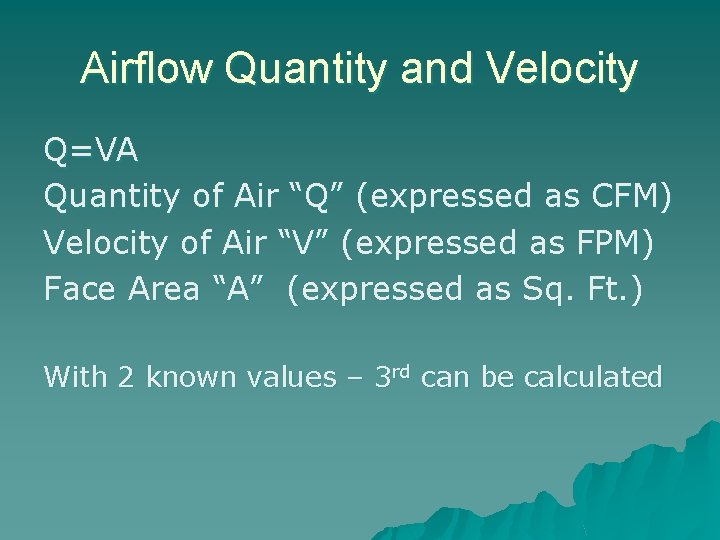Airflow Quantity and Velocity Q=VA Quantity of Air “Q” (expressed as CFM) Velocity of Air “V” (expressed as FPM) Face Area “A” (expressed as Sq. Ft. ) With 2 known values – 3 rd can be calculatedCalculating Quantity (CFM) When face area and velocity are known the CFM can be determined. Q=VA 4 x 500 = 2000 4 sq. ft. x 500 FPM = 2000 CFMCalculating Velocity (FPM) When face area and air quantity are known the velocity can be determined. V=Q/A 2000 / 4 = 500 2000 CFM / 4 sq. ft. = 500 FPM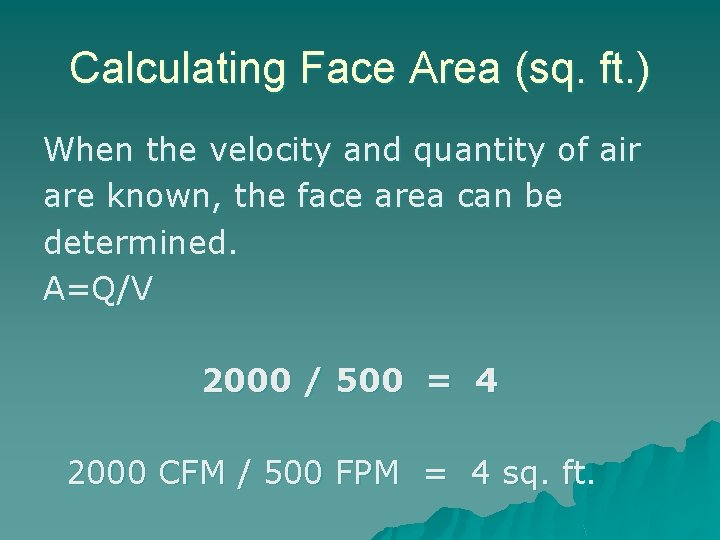Calculating Face Area (sq. ft. ) When the velocity and quantity of air are known, the face area can be determined. A=Q/V 2000 / 500 = 4 2000 CFM / 500 FPM = 4 sq. ft.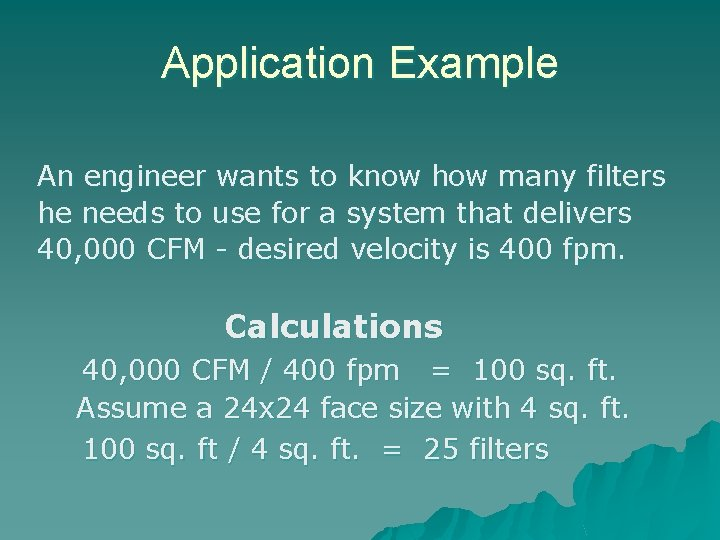Application Example An engineer wants to know how many filters he needs to use for a system that delivers 40, 000 CFM - desired velocity is 400 fpm. Calculations 40, 000 CFM / 400 fpm = 100 sq. ft. Assume a 24 x 24 face size with 4 sq. ft. 100 sq. ft / 4 sq. ft. = 25 filters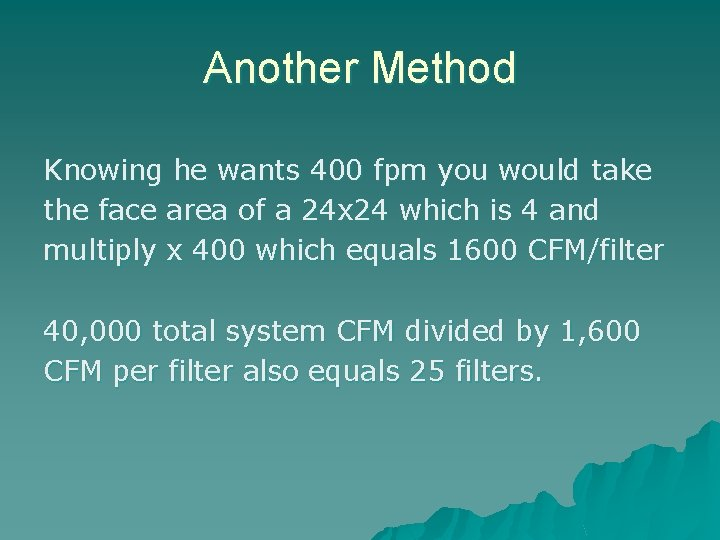Another Method Knowing he wants 400 fpm you would take the face area of a 24 x 24 which is 4 and multiply x 400 which equals 1600 CFM/filter 40, 000 total system CFM divided by 1, 600 CFM per filter also equals 25 filters.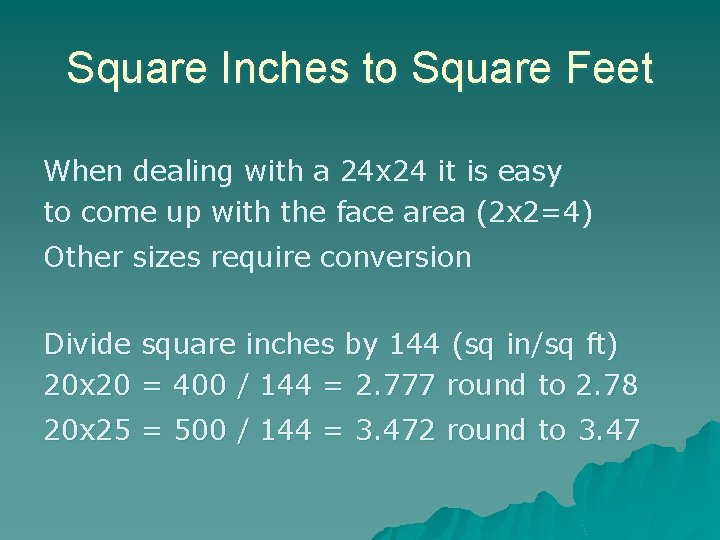Square Inches to Square Feet When dealing with a 24 x 24 it is easy to come up with the face area (2 x 2=4) Other sizes require conversion Divide square inches by 144 (sq in/sq ft) 20 x 20 = 400 / 144 = 2. 777 round to 2. 78 20 x 25 = 500 / 144 = 3. 472 round to 3. 47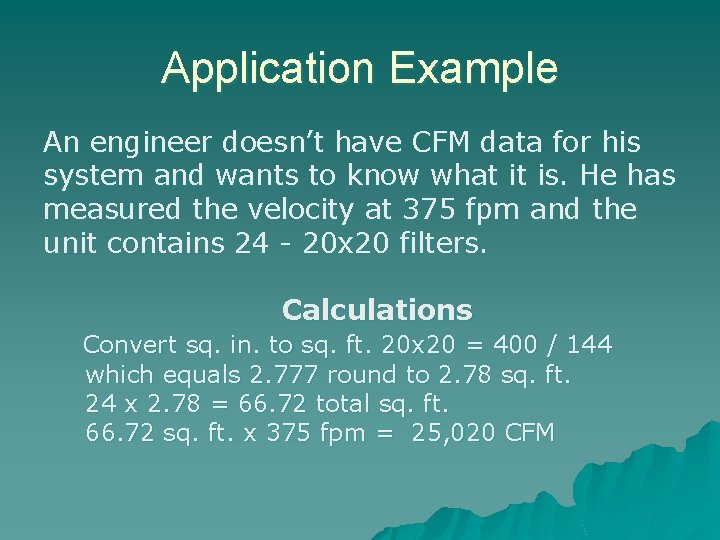Application Example An engineer doesn’t have CFM data for his system and wants to know what it is. He has measured the velocity at 375 fpm and the unit contains 24 - 20 x 20 filters. Calculations Convert sq. in. to sq. ft. 20 x 20 = 400 / 144 which equals 2. 777 round to 2. 78 sq. ft. 24 x 2. 78 = 66. 72 total sq. ft. 66. 72 sq. ft. x 375 fpm = 25, 020 CFM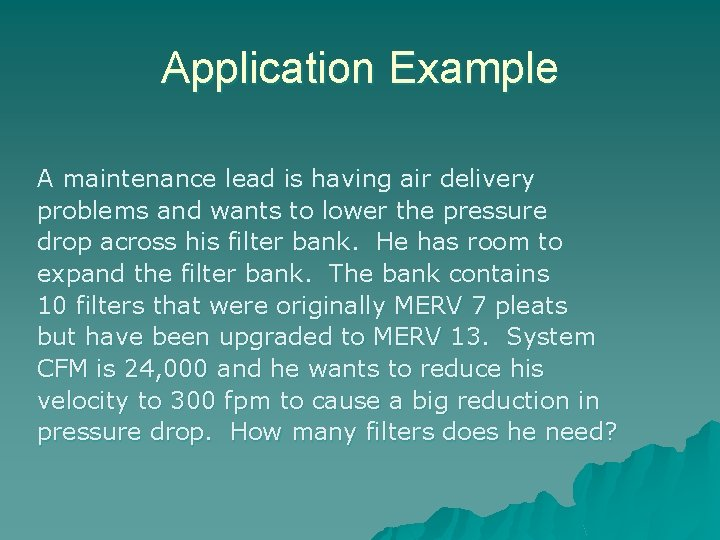Application Example A maintenance lead is having air delivery problems and wants to lower the pressure drop across his filter bank. He has room to expand the filter bank. The bank contains 10 filters that were originally MERV 7 pleats but have been upgraded to MERV 13. System CFM is 24, 000 and he wants to reduce his velocity to 300 fpm to cause a big reduction in pressure drop. How many filters does he need?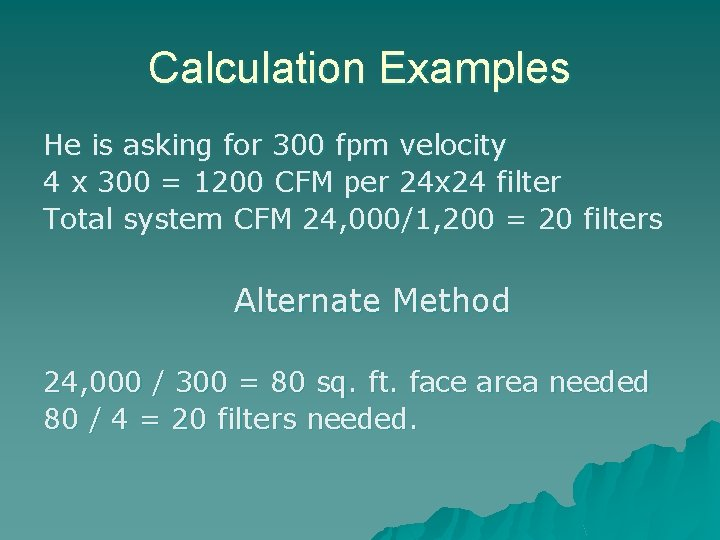Calculation Examples He is asking for 300 fpm velocity 4 x 300 = 1200 CFM per 24 x 24 filter Total system CFM 24, 000/1, 200 = 20 filters Alternate Method 24, 000 / 300 = 80 sq. ft. face area needed 80 / 4 = 20 filters needed.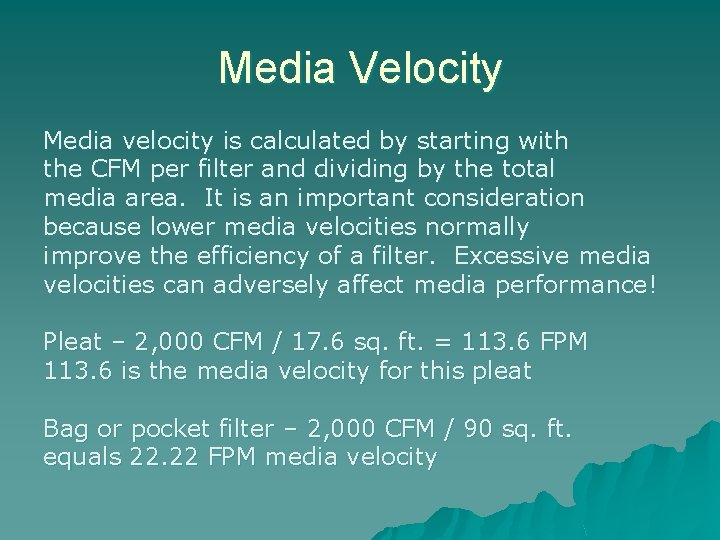Media Velocity Media velocity is calculated by starting with the CFM per filter and dividing by the total media area. It is an important consideration because lower media velocities normally improve the efficiency of a filter. Excessive media velocities can adversely affect media performance! Pleat – 2, 000 CFM / 17. 6 sq. ft. = 113. 6 FPM 113. 6 is the media velocity for this pleat Bag or pocket filter – 2, 000 CFM / 90 sq. ft. equals 22. 22 FPM media velocityAdditional Examples Box – 2, 000 CFM / 58 sq. ft. = 34. 48 FPM Cell – 2, 000 CFM / 100 sq. ft. = 20 FPM 4 V – 2, 000 CFM / 194 sq. ft. = 10. 31 FPM Hepa – 1, 000 CFM / 160 sq. ft. = 6. 25 FPM